# AP Physics C: Electricity and Magnetism Practice Test 7

### Test Information11 questions15 minutes

1. Which of Maxwell's equations allows for the calculation of a magnetic field due to a changing electric field?

2.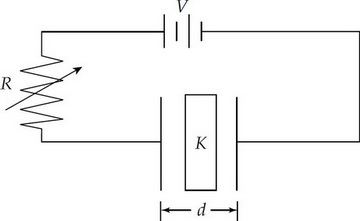A parallel plate capacitor has a dielectric material between the plates with a constant K. The capacitor is connected to a variable resistor R and a power supply of potential difference V. Each plate of the capacitor has a cross-sectional area A and the plates are separated by a distance d. Which of the following changes could increase the capacitance and decrease the amount of charge stored on the capacitor?

3.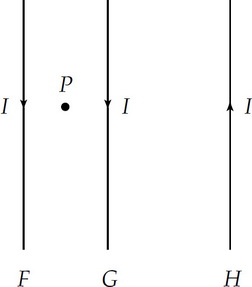Three parallel wires, F, G, and H, all carry equal current I, in the directions shown above. Wire G is closer to wire F than to wire H. The magnetic field at point P is directed

4. A solid, metal object is isolated from other charges and has charge distributed on its surface. The charge distribution is not uniform. It may be correctly concluded that the

5. Question below relates to the circuit represented below. The switch S, after being open a long time, is then closed.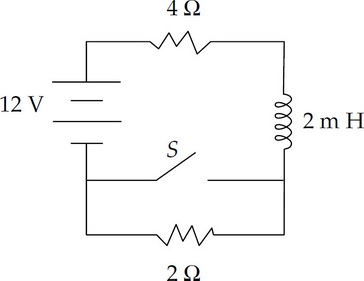What is the potential difference across the inductor immediately after the switch is closed?

6. Question below relates to the circuit represented below. The switch S, after being open a long time, is then closed.What is the current through the 4 Ω resistor after the switch has been closed a long time?

7. A spherical charge distribution varies with the radius r by the equation

ρ = ar, where ρ is the volume charge density and a is a positive constant. The distribution goes out to a radius R.

Which of the following is true of the electric field strength due to this charge distribution at a distance r from the center?

8.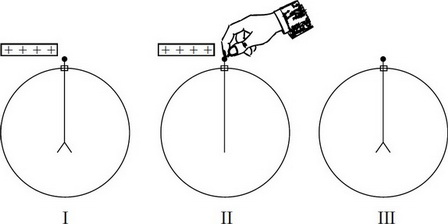When a positively charged rod is brought near, but does not touch, the initially neutral electroscope shown above, the leaves repel (I). When the electroscope is then touched with a finger, the leaves hang vertically (II). Next when the finger and finally the rod are removed, the leaves repel again (III). During the process shown in Figure II

9.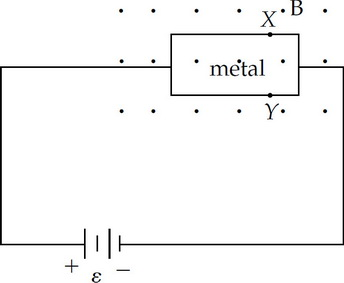A piece of metal in the plane of the page is connected in a circuit as shown above, causing electrons to move through the metal to the left. The piece of metal is in a magnetic field B directed out of the page. X and Y are points on the edge of the metal. Which of the following statements is true?

10. Experimenter A uses a very small test charge qo, and experimenter B uses a test charge 2qo to measure an electric field produced by two parallel plates. A finds a field that is

11. A solid conducting sphere has radius R and carries positive charge Q. Which of the following graphs represents the electric field E as a function of the distance r from the center of the sphere?